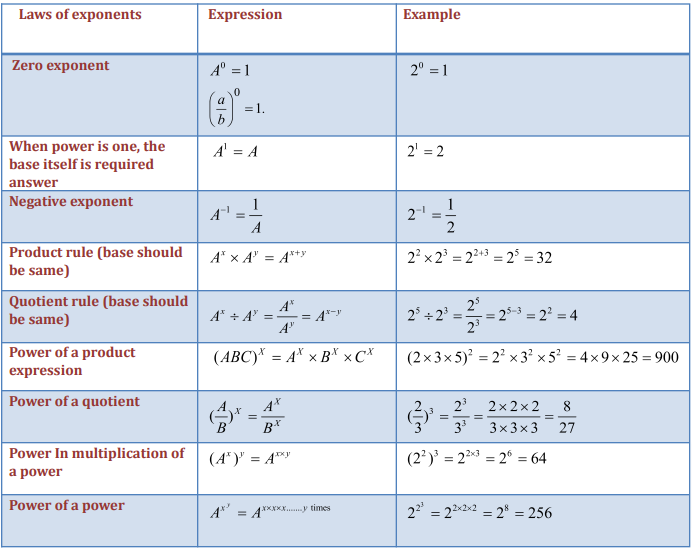Important Laws of Exponents and Related Properties

In the first article for this lesson, we essentially covered the basic concepts for exponents. In this article, we cover the essential laws that govern this topic. Remember, it is vital for you to learn and memorize these rules as most questions for this topic are based on these rules.

Also, when you are learning these rules, make sure you derive these rules. Most of these rules are based on common sense and you should be able to understand the logic operating behind these laws of exponents.

Go through the following table to learn the various rules:Examples using the Laws of Exponents:

Let us take some examples to make the rules mentioned above clearer.

Example 1:  EvaluateSolution:Example 2: EvaluateSolution:

We know thatSo,Example 3: EvaluateSolution:

We know thatsoExample 4: EvaluateSolution:We have

==Example 5: By what number shouldbe multiplied so that the product isSolution:

Let the required number be x. Then,Hence, the required number is.

Comparison of exponents

An important question type that is based on this topic is related to comparison of exponents. So how can we compare two different exponents? Let us go through some rules that you can memorize to solve these directly and in the shortest possible time.

Case-1: When the power is sameLet us take an example question to understand the concept above.

Example: Which is greater?

Solution:

As we know that 2 < 3

SoCase-2: When the bases are sameLet us take an example question to understand the concept above.

Example: Which is greater?

Solution:Case-3: When both the power and based are different

In this case, we essentially have two numbers:Let us understand this with an example:

Example: Which is greater?

Solution:Let us take one more example to make this concept clearer.

Example: Which is greater?

Solution:EXERCISE:

Question 1: Evaluatea. 1/27

b. 1/9

c. 1

d. 27

Solution: option aQuestion 2: Evaluatea. 16/81

b. 81/16

c. 3/16

d. 9/16

Solution: option b

We know thatSo,Question : Evaluatea. 8/729

b. 8

c. -729/8

d. -8/729

Solution: option a

We know thatsoQuestion 4: Evaluatea.100/49

b. 100/147

c. 100

d. 147/49

Solution: option bQuestion 5: By what number shouldbe multiplied so that the product isa. 16/15

b. -15/16

c. -16/15

d. -15/16

Solution: option c

Let the required number be x. Then,